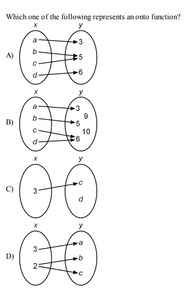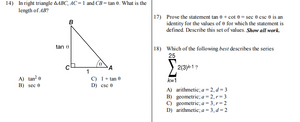# Algebra 2 Common Core

Regular price \$12.95 SaleSubscription details
How subscriptions work

Products are automatically delivered on your schedule. No obligation, modify or cancel your subscription anytime.

Number of Questions: 5,079
Number of Graphics: 1,241
Question Format: Multiple choice, free response, true/false, and multi-step

Examgen's new Common Core Algebra II database is a comprehensive question bank created to support the common core requirements that will allow students to build new mathematical knowledge through a variety of problem-solving strategies. The question bank includes all required topics for the Common Core curriculum with emphasis on function theory, algebraic concepts, and statistics. Verbal, numeric, graphical, and algebraic approaches enable students to apply acquired knowledge to solve problems.

Here are some of the topics covered in the Common Core Algebra II:

• Polynomial Factoring
• Polynomial Equations, Inequalities, and Functions
• Real World Applications of Rational Expressions
• Radical Expression: Products and Conjugates
• Factors with Irrational Coefficients
• Complex Numbers Represented as Points
• Polynomial Equations with Complex Roots
• Systems of Two Quadratic Equations
• Writing Equations of Parabolas
• Function Notation: Average Rate of Change
• Exponential Functions, Equations, Transformations
• Circular Trigonometry: Reference Angles
• Sketching Sine and Cosine Functions
• Probability Rules
• Statistical Studies and Sampling Distributions

More about the Questions Bank and Test Generator